Learning Library
premium

# 5.NBT.B.7 Worksheets, Workbooks, Lesson Plans, and Games

#### CCSS.MATH.CONTENT.5.NBT.B.7

:
"Add, subtract, multiply, and divide decimals to hundredths, using concrete models or drawings and strategies based on place value, properties of operations, and/or the relationship between addition and subtraction; relate the strategy to a written method and explain the reasoning used."

These worksheets can help students practice this Common Core State Standards skill.

## Worksheets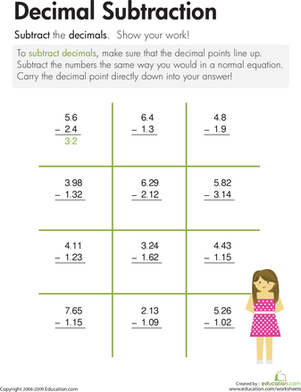Decimal Subtraction
Worksheet
Decimal Subtraction
Let's get to the point: the decimal point, that is! On this third grade math worksheet, kids learn how to subtract decimals.
3rd grade
Math
Worksheet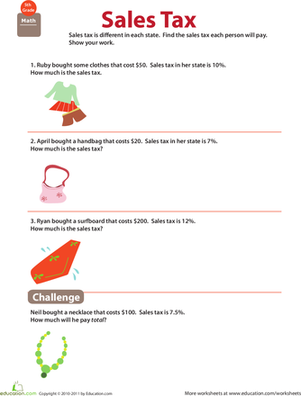Money Math: Solve Sales Tax Word Problems
Worksheet
Money Math: Solve Sales Tax Word Problems
This worksheet's focus on sales tax gives your fifth grader practice with multiplication and decimals.
5th grade
Worksheet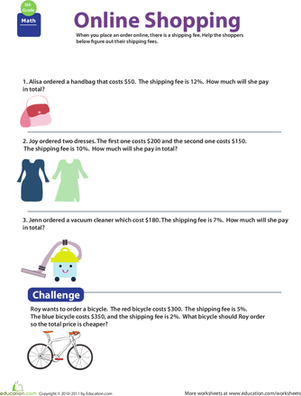Money Math: Shopping and Shipping #1
Worksheet
Money Math: Shopping and Shipping #1
Teach your kid about a not-so-fun fact of life: shipping fees, and improve his ability to multiply decimals, with this colorful worksheet.
5th grade
Worksheet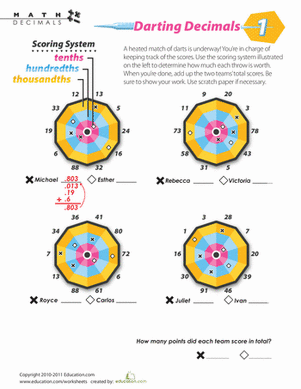Darting Decimals #1
Worksheet
Darting Decimals #1
In this decimal dart game, your kid plays scorekeeper as he adds up the decimals and determines the winner.
5th grade
Math
Worksheet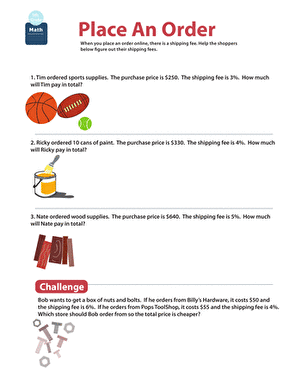Money Math: Shopping and Shipping #2
Worksheet
Money Math: Shopping and Shipping #2
Can your fifth grader use multiplication to figure out the shipping fees for each word problem?
5th grade
Worksheet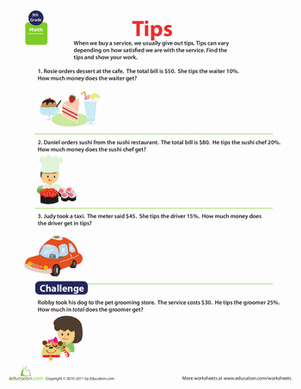Money Math: Calculate Tips
Worksheet
Money Math: Calculate Tips
Tips are a big part of real life, and they provide a perfect opportunity to practice fifth grade math!
5th grade
Worksheet

## Lesson PlansDividing Decimals Dash!
Lesson plan
Dividing Decimals Dash!
Get students moving in this fun lesson that involves a relay race style game! This lesson focuses on dividing decimals by whole numbers.
5th grade
Math
Lesson plan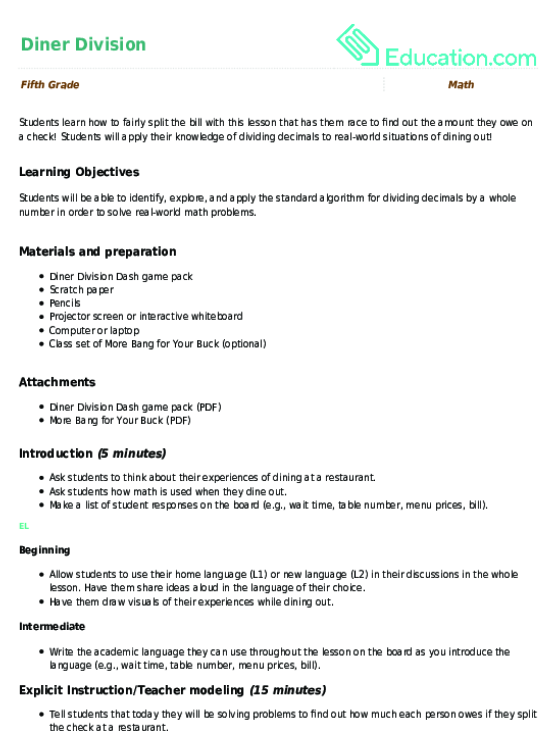Diner Division
Lesson plan
Diner Division
Students learn how to fairly split the bill with this lesson that has them race to find out the amount they owe on a check! Students will apply their knowledge of dividing decimals to real-world situations of dining out!
5th grade
Math
Lesson planDecimals, Decimals, Decimals!
Lesson plan
Decimals, Decimals, Decimals!
This lesson combines three essential skills in one entertaining game! Students will need to add, subtract, and understand place value with decimals.
5th grade
Math
Lesson planSurvey Says...
Lesson plan
Survey Says...
This hands-on lesson covers fractions and percentages. Children will have a great time interacting with their fellow classmates and completing surveys as they learn to represent parts of a whole.
5th grade
Math
Lesson planCandy Math: All About Decimals
Lesson plan
Candy Math: All About Decimals
Help students add and subtract decimals in this visual, hands-on, and delicious lesson! This lesson also reinforces place value concepts with decimals.
5th grade
Math
Lesson planMoney Matters
Lesson plan
Money Matters
Take your students on a virtual shopping trip to allow them to explore the importance of spending money wisely.
5th grade
Math
Lesson plan

## Workbooks

No workbooks found for this common core node.

## Games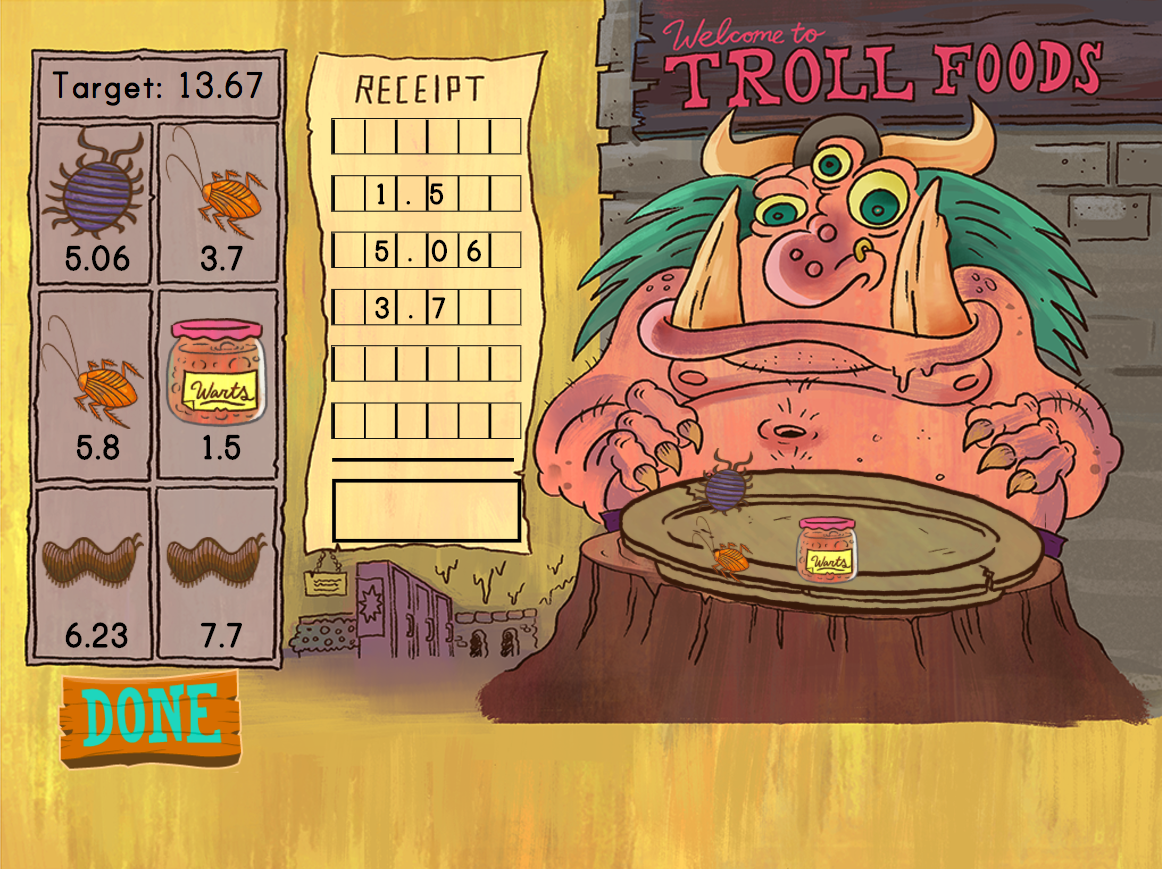Addition with Decimals: Silly Salad Weights
Game
Addition with Decimals: Silly Salad Weights
Fe, fi, fo, yum! Kids practice decimal addition to the tenth and hundredth place with this silly game.
5th grade
Math
Game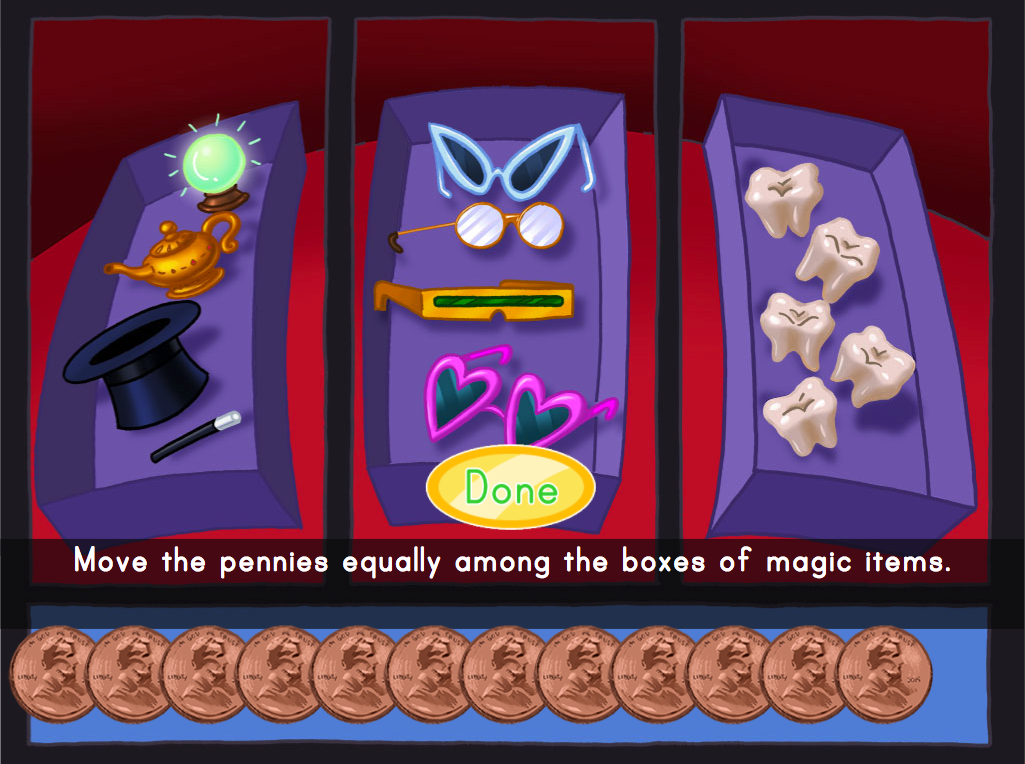Decimal Division with Pennies
Game
Decimal Division with Pennies
Kids practice decimal division to the hundredths by working with pennies in this interactive shopping game.
5th grade
Math
GameRecord-Breaking Penelope
Story
Record-Breaking Penelope
Penelope breaks silly world records in this interactive math story.
5th grade
Math
Story

## Exercises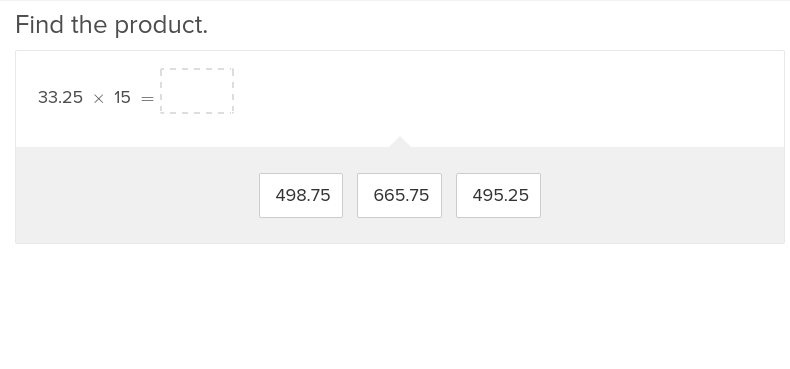Multiplying Decimal Numbers to the Hundredths Place
Exercise
Multiplying Decimal Numbers to the Hundredths Place
Build on your students' knowledge of decimals and multiplication with these exercises that incorporate both skills together.
5th grade
Math
Exercise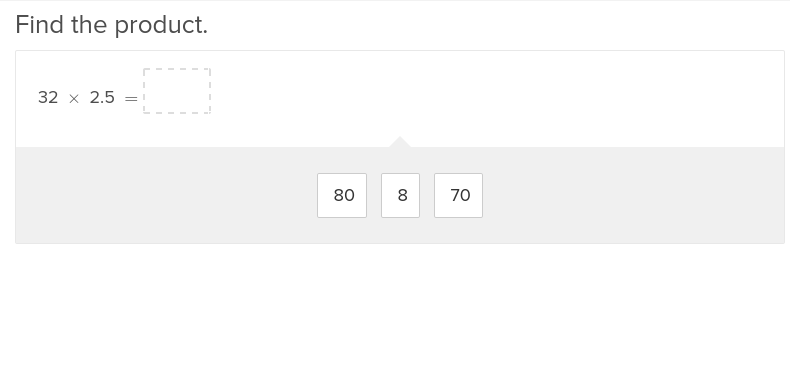Multiplying Decimal Numbers to the Tenths Place
Exercise
Multiplying Decimal Numbers to the Tenths Place
Teach your fifth grader the importance of multiplying by decimals with these exercises that give them actual situations and helpful hints.
5th grade
Math
Exercise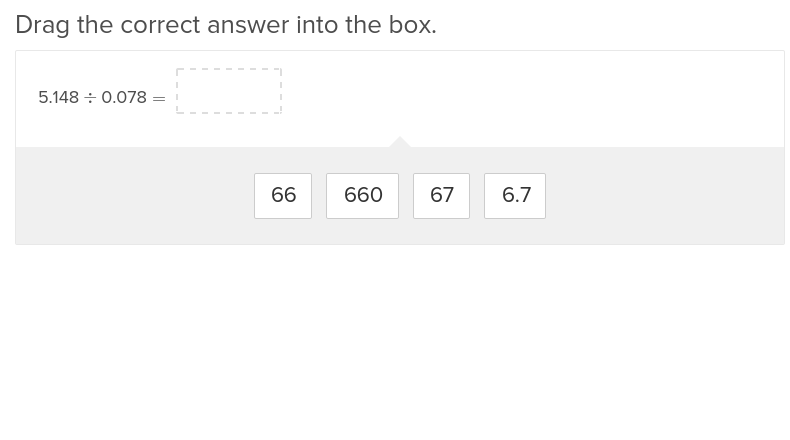Multi-Digit Decimal Division
Exercise
Multi-Digit Decimal Division
Once students have mastered decimal division to the tenths place, elevate their skills with this exercise on multi digit decimal division.
5th grade
Math
Exercise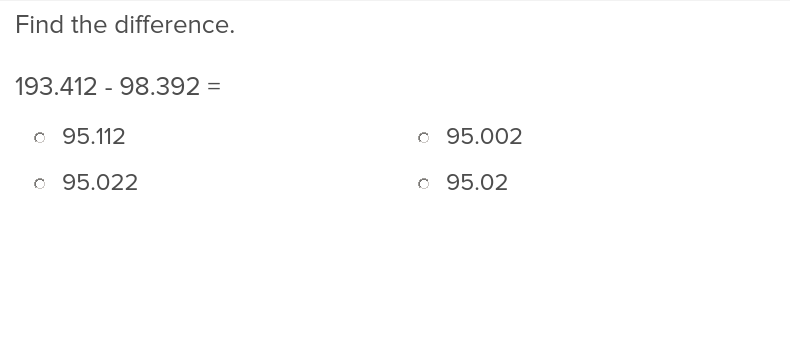Subtraction with Decimal Numbers to the Thousandths Place
Exercise
Subtraction with Decimal Numbers to the Thousandths Place
This exercise on subtraction to the thousandths place will fine tune students’ attention to detail.
5th grade
Math
ExerciseAddition with Decimal Numbers to the Thousandths Place
Exercise
Addition with Decimal Numbers to the Thousandths Place
Showing students how to add decimal numbers to the thousandths is a snap when you take advantage of this easy to use exercise.
5th grade
Math
Exercise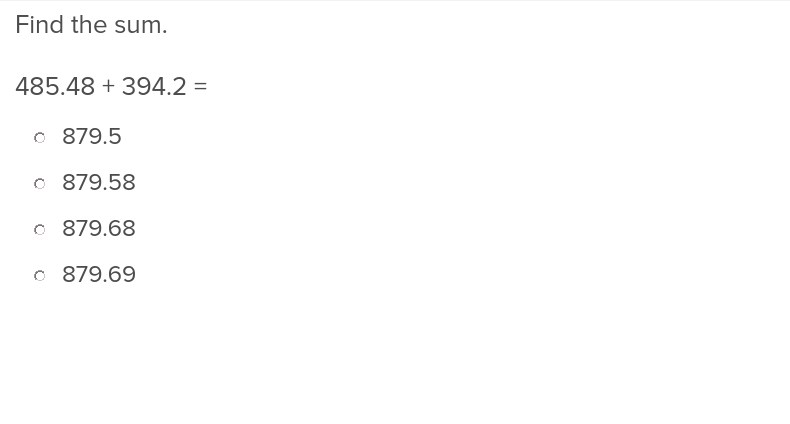Addition with Decimal Numbers to the Hundredths Place
Exercise
Addition with Decimal Numbers to the Hundredths Place
Enhancing students’ competency with decimal numbers to the hundredths place is a simple matter of assigning them this exercise.
5th grade
Math
Exercise

### Add to collection

Create new collection

0

### New Collection>

0 items

What could we do to improve Education.com?

Please note: Use the Contact Us link at the bottom of our website for account-specific questions or issues.

What would make you love Education.com?